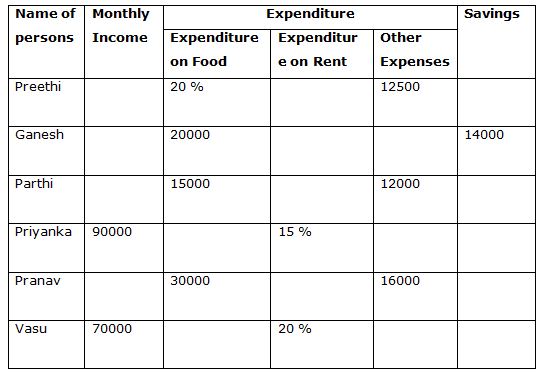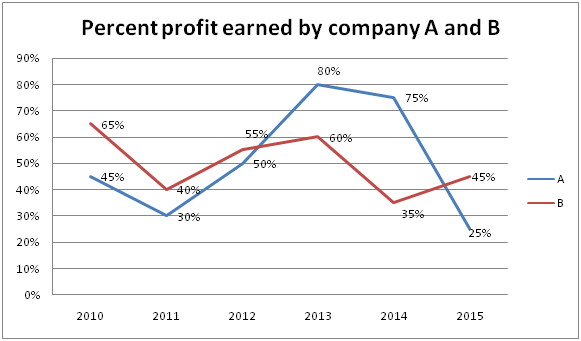# Quantitative Aptitude Questions (Data Interpretation) for RRB PO Mains 2018 Day-188

Dear Readers, IBPS RRB is conducting Online Examination for the recruitment of Assistant. To enrich your preparation here we have providing new series of Data Interpretation – Quantitative Aptitude Questions. Candidates those who are appearing in RRB PO  Mains Exams can practice these Quantitative Aptitude average questions daily and make your preparation effective.

[WpProQuiz 3393]

Click “Start Quiz” to attend these Questions and view Solutions

Directions (1 – 5): Study the following information carefully and answer the given questions.

The following table shows the Income, Expenditure and Savings of 6 different persons. Some data’s are missing.1) If the total amount paid by Ganesh and Parthi for Rent is equal to Rs. 60000 and the other expenses of Ganesh is 20% of his monthly income, which is equal to Rs. 100000 then find the savings of Parthi if the expenditure on Rent is 25% of his income?

a) Rs. 18000

b) Rs. 21000

c) Rs. 15000

d) Rs. 24000

e) None of these

2) If the ratio between the savings of Ganesh and Pranav is 4 : 5 and the amount spent on rent by Pranav is 50% of his income, then find the new savings of Pranav if his monthly expenditure on food increases by 10% and other expenses remains the same?

a) Rs. 17500

b) Rs. 19000

c) Rs. 18000

d) Rs. 14500

e) None of these

3) If the savings of Priyanka is 20% less than the saving of Ganesh and the ratio of her expenditure on food and other expenses is 7 : 3, then find the approximate percentage expenditure on food out of her monthly income?

a) 15 %

b) 30 %

c) 51 %

d) 35 %

e) 40 %

4) If 40 % of the amount spent on food by Vasu is equal to Rs. 5000 and his other expenses is 50% more than his savings, then find the ratio between his expenditure on rent to that of savings?

a) 25 : 32

b) 21 : 17

c) 15 : 19

d) 70 : 87

e) 56 : 63

5) If the sum of other expenses, rent and savings of Preethi is Rs. 60000, then the Preethi’s expenditure on food is what percentage more/less than the Preethi’s expenditure on other expenses?

a) 25 % more

b) 20 % more

c) 15 % less

d) 20 % less

e) 15 % more

Directions (6 – 10): Study the following information carefully and answer the given questions.

The following line graph shows the percentage profit earned by two companies A and B in six different years.6) If the expenditure of company A in 2010 is Rs. 60000 and that of company B in 2013 is Rs. 150000, then find the sum of their incomes?

a) Rs. 352000

b) Rs. 327000

c) Rs. 315000

d) Rs. 298000

e) None of these

7) If the incomes of company A and company B are same in 2014, then find the ratio of their expenditures?

a) 7 : 5

b) 27 : 35

c) 16 : 25

d) 3 : 7

e) None of these

8) Total percentage profit earned by company A in all the given years is approximately what percentage of total percentage profit earned by company B in all the given years?

a) 115 %

b) 94 %

c) 102 %

d) 83 %

e) 75 %

9) If the expenditure of company A and B in 2012 are Rs. 75000 and Rs. 120000 respectively, then the income of company B is approximately what percentage more than the income of company A in 2012?

a) 70 %

b) 50 %

c) 60 %

d) 65 %

e) 75 %

10) Which of the following statement is true about company A?

a) Profit earned by company A is maximum in 2013

b) Profit earned by company A is minimum in 2015

c) Profit earned by company A is more than that of company B in 2011

d) Can’t be determined

e) None of these

Direction (1-5) :

Other expenses of Ganesh = 100000*(20/100) = Rs. 20000

Expenditure on rent by Ganesh

= > 100000 – (20000 + 14000 + 20000) = Rs. 46000

Rent expenditure of Parthi = 60000 – 46000 = Rs. 14000

Monthly Income of Parthi = 14000*(100/25) = Rs. 56000

Savings of Parthi = 56000 – (14000 + 15000 + 12000) = Rs. 15000

Savings of Pranav= 14000*(5/4) = Rs. 17500

Let the monthly income of Pranav be x,

According to the question,

17500 + 30000 + 16000 + 50/100 of x = x

63500 + 5x/10 = x

63500 = x – 5x/10

63500 = 5x/10

x= 63500*(10/5)

x= Rs. 127000

Rent paid by Pranav = 127000*(50/100) = Rs. 63500

Expenditure on food by Pranav after increase = 30000*(110/100) = Rs. 33000

New savings of Pranav = 127000 – (33000 + 63500 + 16000) = Rs. 14500

Savings of Priyanka= 14000*(80/100) = Rs. 11200

Rent expenditure of Priyanka = 90000*(15/100) = Rs. 13500

Total expenditure of Priyanka for food and other expenses,

= > 90000 – (11200 + 13500) = Rs. 65300

Expenditure on food by Priyanka = 65300*(7/10) = Rs. 45710

Required percentage = (45710/90000)*100 = 51 %

Total expenditure on food by Vasu = 5000*(100/40) = Rs. 12500

Rent paid by Vasu = 70000*(20/100) = Rs. 14000

Total expenditure on other expenses and savings of Vasu

= > 70000 – (14000 + 12500) = Rs. 43500

Other expenses = (150/100)*savings

The ratio of other expenses to that of savings of Vasu = 3 : 2

5’s = 43500

1’s = 8700

The savings of Vasu = 8700*2 = Rs, 17400

Required ratio = 14000 : 17400= 70 : 87

Expenditure on food of Preethi = 20 %

The sum of other expenses, rent and savings of Preethi = Rs. 60000

80 % of total income of Preethi = 60000

Preethi’s income = 60000*(100/80) = Rs. 75000

Expenditure on food of Preethi = 75000*(20/100) = Rs. 15000

Expenditure on other expenses of Preethi = Rs. 12500

Required percentage = [(15000 – 12500)/12500]*100 = 20 % more

Direction (6-10) :

Income of company A in 2010 = 60000*(145/100) = Rs. 87000

Income of company B in 2013 = 150000*(160/100) = Rs. 240000

Required sum = 87000 + 240000 = Rs. 327000

Let income of company A and B be Rs. x in 2014,

Income (A) = exp (A)*(175/100)

Exp (A) = income (A)*(100/175) = x*(100/175)

Income (B) = exp (B)*(135/100)

Exp (B) = income (B)*(100/135) = x*(100/135)

Exp (A) : Exp (B) = (x*100/175) : (x*100/135) = 135 : 175 = 27 : 35

Total percent profit earned by company A over all the years

= > 45 + 30 + 50 + 80 + 75 + 25 = 305

Total percent profit earned by company B over all the years

= > 65 + 40 + 55 + 60 + 35 + 45 = 300

Required percentage = (305/300)*100 = 101.67 % = 102 %

Income of company A in 2012 = 75000*(150/100) = Rs. 112500

Income of company B in 2012 = 120000*(155/100) = Rs. 186000

Required percentage = [(186000 – 112500)/112500]*100

= > [73500/112500]*100 = 65.33% = 65 %

We cannot say profit earned by company is more or less in any year, because only profit % is given and without knowing the expenditure/Income, profit cannot be determined.

Daily Practice Test Schedule | Good Luck

 Topic Daily Publishing Time Daily News Papers & Editorials 8.00 AM Current Affairs Quiz 9.00 AM Quantitative Aptitude “20-20” 11.00 AM Vocabulary (Based on The Hindu) 12.00 PM General Awareness “20-20” 1.00 PM English Language “20-20” 2.00 PM Reasoning Puzzles & Seating 4.00 PM Daily Current Affairs Updates 5.00 PM Data Interpretation / Application Sums (Topic Wise) 6.00 PM Reasoning Ability “20-20” 7.00 PM English Language (New Pattern Questions) 8.00 PM